# Classes and Objects in JavaScript

ECMAScript 5 does not have the explicit concept of Class. Instead, it simulates a class based on the two concepts such as function & prototype. Because ES5 was introduced in 2011, its syntax has been very popular so far; however, we can not deny that creating a class in such a way is hard to understand for developers. Meanwhile, the languages such as Java, C#, .. have a more modern way to create a class. The ES6 version, released in 2015, promptly patches this issue. It provides a modern syntax for defining a class.
Below, I have a Rectangle, which has 2 important properties such as width and height. We will define a class named Rectangle to simulate it with the ES6 syntax.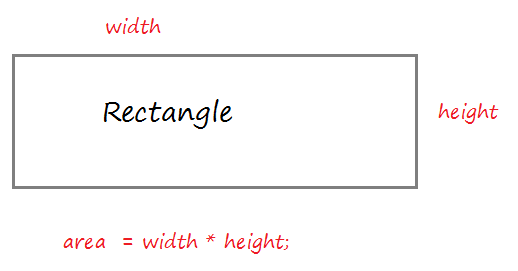Create a resource file rectangle.js:
rectangle.js
``````// Define a class.
class Rectangle  {

// Contructor with 2 parameters.
// (Used to create instance)
// this.width refers to the width property of the class
constructor (width = 5 , height = 10)  {
this.width = width;
this.height = height;
}

// A method calculates the area of the rectangle.
getArea() {
var area = this.width * this.height
return area
}

}

// Create an object of Rectangle via Constructor.
var rect = new Rectangle(3, 5);

console.log("Height "+ rect.height);
console.log("Width "+ rect.width);

// Call method
let area = rect.getArea();
console.log("Area "+ area );``````
Running the example:
``````Height 5
Width 3
Area 15``````
What will happen when you create an object from the constructor of the class?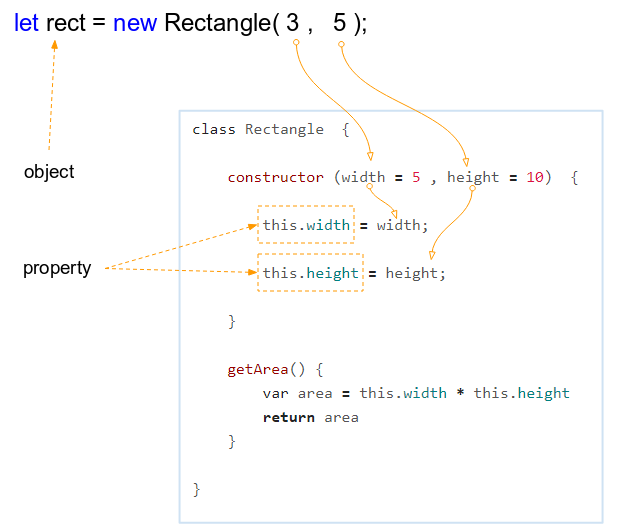When you callthe Constructor of class, a new object will be created and the properties of the object will be assigned values from parameters.
In the ECMAScript, each class has at most only one constructor. Like a function, the parameters of the constructor can also have a default value. So you can create objects in different ways.
``````// width = 3, height = 5
let rect = new Rectangle(3, 5);

// width = 15, height = 10 (Default)
let rect2 = new Rectangle(15);

// width = 5 (Default), height = 50
let rect3 = new Rectangle(undefined, 50);

// width = 5 (Default), height = 10 (Default)
let rect4 = new Rectangle();``````

## 2. Getter & Setter

Before giving the concepts of Getter & Setter, let's analyze a situation:
Assume that we have a Person class and this class has a property which is name.
Class Person
``````class Person  {

constructor(name) {
this.name = name;
}
}``````
And you can access the properties of the object, or assign a new value to it without having any problems.
``````// Create an object
let person = new Person("Tom");

console.log( person.name); // Tom

// Assign new value to property name.
person.name = "Jerry"; // !!!

console.log( person.name ); // Jerry``````
Accessing freely to a property and changing its value from the outside of the class are really dangerous. Sometimes, you want to have a property that it is not possible to access, or assign a new value to from the outside of the class. Getter/Setter allows you to create such a property.
Okay, we write the Person class again. get keyword put in front of a method without the class parameters helps create a property with the name which is the method name. This method will be called every time when the program accesses this property.
getter-example1.js
``````class Person  {

constructor (name)  {
// property: __name
this.__name = name;
}

// Getter of property name
get name()  {
console.log("Call getter of property 'name'!!");
return this.__name;
}

}

// ------------ TEST -----------------

let person = new Person("Tom");

console.log( person.name); // Tom

// Assign new value to property name.
person.name = "Jerry"; // Not Working!!!!

console.log( person.name); // Tom``````
set keyword put in front of a method with one parameter of class helps create a property with the name which is the method name. This method will be called everytime the program assigns a new value to this property.
setter-example1.js
``````class Person  {

constructor (name)  {
// property: __name
this.__name = name;
}

// Setter of property name
set name(newName)  {
console.log("Call setter of property 'name'!!");
this.__name = newName;
}

// A method
showInfo()  {
console.log("Person: " + this.__name);
}
}

// ------------ TEST -----------------

let person = new Person("Tom");

console.log( person.name); // undefined

// Set new value to property 'name' ==> Call setter
person.name = "Jerry";

person.showInfo(); // Person: Tom``````
Example of a property with Getter & Setter:
getter-setter-example.js
``````class Rectangle  {

constructor (width = 5 , height = 10)  {
this.__width = width;
this.height = height;
}

// Getter of property 'width'
get width()  {
return this.__width;
}

// Setter of property 'width'
set width(newWidth)  {
if(newWidth > 0) {
this.__width = newWidth;
} else {
console.log("Invalid width " + newWidth);
}
}

}

// ------------ TEST ------------------

var rect = new Rectangle(3, 5);

console.log("Height "+ rect.height); // Height: 5
console.log("Width "+ rect.width); // Width: 3

rect.width = -100;

console.log("Height "+ rect.height); // Height: 5
console.log("Width "+ rect.width); // Width: 3

rect.width = 100;

console.log("Height "+ rect.height); // Height: 5
console.log("Width "+ rect.width); // Width: 100``````
Output:
``````Height 5
Width 3
Invalid width -100
Height 5
Width 3
Height 5
Width 100``````
The properties with prefixes: 2 underscores (__) are usually conventionalized by programmers not to use it outside the class. However, that convention can be broken by someone. And therefore, using such properties is dangerous.
If you want a private property, it should be named with the prefix which is hashtag (#). However, this code can only be run with the help of Babel 7 or later.
Private property
``````class Something {

constructor(){

this.#someProperty = "test";
}

}

const instance = new Something();

console.log(instance.#someProperty); // undefined``````
getter-example2.js
``````class Person  {

constructor (name)  {
// Private property: #name
this.#name = name;
}

// Getter of property name
get name()  {
console.log("Call getter of property 'name'!!");
return this.#name;
}

}``````

## 3. Static Field

The static keyword appears in the Getter or Setter declarations to help you define a static field. You can access static fields through a class name.
static fields with fixed value (unchangeable) are called constant static fields.
static-field-example1.js
``````class Employee {

constructor (fullName, age)  {
this.fullName = fullName;
if(age < Employee.MIN_AGE || age > Employee.MAX_AGE)  {
throw "Invalid Age " + age;
}
this.age = age;
}

// A static field: MIN_AGE
static get MIN_AGE() {
return 18;
}

// A static field: MAX_AGE
static get MAX_AGE() {
if(!Employee.__MAXA)  {
Employee.__MAXA = 60;
}
return Employee.__MAXA;
}

static set MAX_AGE(newMaxAge)  {
Employee.__MAXA = newMaxAge;
}

}

// ---- TEST ---------

console.log("Mininum Age Allowed: " + Employee.MIN_AGE);
console.log("Maximum Age Allowed: " + Employee.MAX_AGE);

// Set new Maximum Age:
Employee.MAX_AGE = 65;

console.log("Maximum Age Allowed: " + Employee.MAX_AGE);

let baby = new Employee("Some Baby", 1); // Error!!``````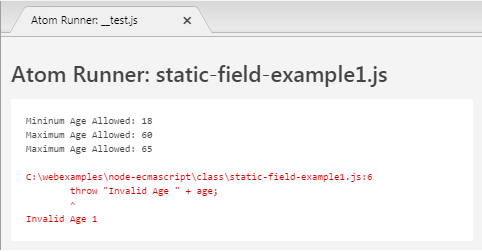You have another way for declaring a static field for a class, however, static fields created by this way will not be a Constant because its value can change. Below is an example:
static-field-example2.js
``````class Employee {

constructor (fullName, age)  {
this.fullName = fullName;
if(age < Employee.MIN_AGE || age > Employee.MAX_AGE)  {
throw "Invalid Age " + age;
}
this.age = age;
}
}

Employee.MIN_AGE = 18;
Employee.MAX_AGE = 60;

// ---- TEST ---------

console.log("Mininum Age Allowed: " + Employee.MIN_AGE);
console.log("Maximum Age Allowed: " + Employee.MAX_AGE);

// Set new Maximum Age:
Employee.MAX_AGE = 65;

console.log("Maximum Age Allowed: " + Employee.MAX_AGE);

let baby = new Employee("Some Baby", 1); // Error!!``````

## 4. Static method

The Static keyword which appears in declaration of a class method helps you define a static method. You can call static methods through the class name. Static methods can not be called through the object of the class. A static method is often used as an utility function of an application.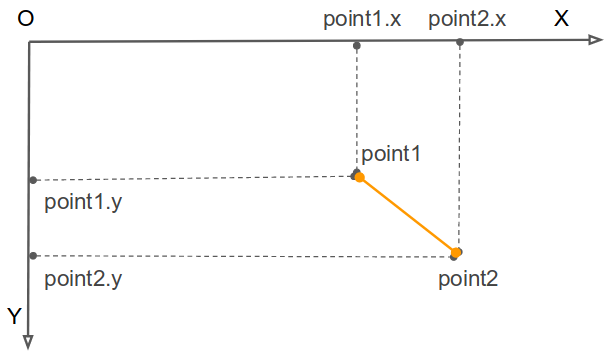point.js
``````class Point {

constructor(x, y) {
this.x = x;
this.y = y;
}

// Calulatesthe distance between 2 points
static distance( point1, point2) {
const dx = point1.x - point2.x;
const dy = point1.y - point2.y;

return Math.hypot(dx, dy);
}
}

// --- TEST ---
let point1 = new Point( 5, 10);
let point2 = new Point( 15, 20);

// Distance
let d = Point.distance(point1, point2);

console.log("Distance between 2 points: " + d);``````
Output:
``Distance between 2 points: 14.142135623730951``

## 5. Object Comparison operators

In ECMAScript, when you create an object through constructor will be a real entity is created in memory, it has a specified address.

An operator assigned AA object by an BB object does not create new entity on the memory, it's just pointing to address of AA to BB's address.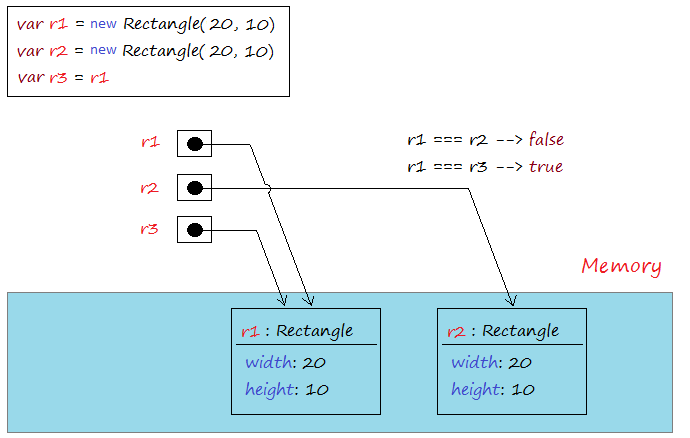=== Operator used to compare two objects that is pointing to, it returns true if the two objects refer to the same address in memory. Operators !== is used to compare the two addresses of two objects that is pointing to, it returns true if the two objects that point to two different addresses.
identify-operator-example.js
``````// Define a class.
class Rectangle  {
constructor (width = 5 , height = 10)  {
this.width = width;
this.height = height;
}
getArea() {
var area = this.width * this.height;
return area;
}
}

// Create object: r1
let r1 = new Rectangle( 20,  10);

// Create object: r2
let r2 = new Rectangle( 20, 10);

let r3 = r1;

let  b12  =   r1 === r2; // false
let  b13  =   r1 === r3; // true

console.log("r1 === r2 ? " + b12); // false
console.log("r1 === r3 ? " + b13); // true

var bb12  = r1 !== r2; // true
var bb13  = r1 !== r3; // false

console.log("r1 !== r2 ? " + bb12); // true
console.log("r1 !== r3 ? " + bb13); // false``````
Running the example:
``````r1 === r2 ? false
r1 === r3 ? true
r1 !== r2 ? true
r1 !== r3 ? false``````